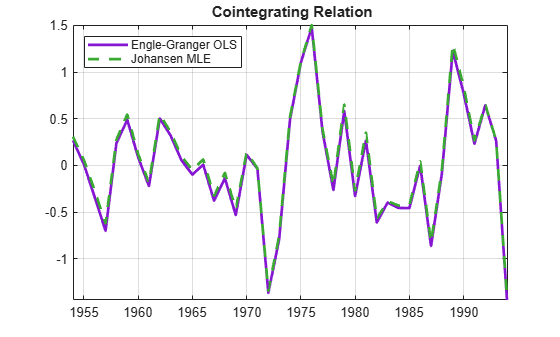Documentation

## Compare Approaches to Cointegration Analysis

Comparing inferences and estimates from the Johansen and Engle-Granger approaches can be challenging, for a variety of reasons. First of all, the two methods are essentially different, and may disagree on inferences from the same data. The Engle-Granger two-step method for estimating the VEC model, first estimating the cointegrating relation and then estimating the remaining model coefficients, differs from Johansen's maximum likelihood approach. Secondly, the cointegrating relations estimated by the Engle-Granger approach may not correspond to the cointegrating relations estimated by the Johansen approach, especially in the presence of multiple cointegrating relations. It is important, in this context, to remember that cointegrating relations are not uniquely defined, but depend on the decomposition $C=A{B}^{\prime }$ of the impact matrix.

Nevertheless, the two approaches should provide generally comparable results, if both begin with the same data and seek out the same underlying relationships. Properly normalized, cointegrating relations discovered by either method should reflect the mechanics of the data-generating process, and VEC models built from the relations should have comparable forecasting abilities.

As the following shows in the case of the Canadian interest rate data, Johansen's H1* model, which is the closest to the default settings of egcitest, discovers the same cointegrating relation as the Engle-Granger test, assuming a cointegration rank of 2:

load Data_Canada Y = Data(:,3:end); % Interest rate data [~,~,~,~,reg] = egcitest(Y,'test','t2'); c0 = reg.coeff(1); b = reg.coeff(2:3); beta = [1; -b]; [~,~,~,~,mles] = jcitest(Y,'model','H1*');
************************ Results Summary (Test 1) Data: Y Effective sample size: 40 Model: H1* Lags: 0 Statistic: trace Significance level: 0.05 r h stat cValue pValue eigVal ---------------------------------------- 0 1 38.8360 35.1929 0.0194 0.4159 1 0 17.3256 20.2619 0.1211 0.2881 2 0 3.7325 9.1644 0.5229 0.0891 
BJ2 = mles.r2.paramVals.B; c0J2 = mles.r2.paramVals.c0; % Normalize the 2nd cointegrating relation with respect to % the 1st variable, to make it comparable to Engle-Granger: BJ2n = BJ2(:,2)/BJ2(1,2); c0J2n = c0J2(2)/BJ2(1,2); % Plot the normalized Johansen cointegrating relation together % with the original Engle-Granger cointegrating relation: h = gca; COrd = h.ColorOrder; plot(dates,Y*beta-c0,'LineWidth',2,'Color',COrd(4,:)) hold on plot(dates,Y*BJ2n+c0J2n,'--','LineWidth',2,'Color',COrd(5,:)) legend('Engle-Granger OLS','Johansen MLE','Location','NW') title('{\bf Cointegrating Relation}') axis tight grid on hold off